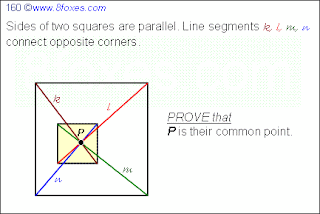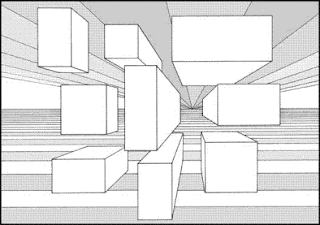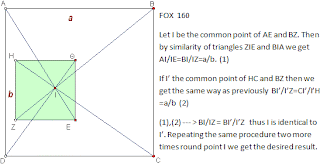Saturday, July 17, 2010

Fox 160 - Solutions

Three distinct solutions were found for Fox 160.The solution commented out by Julian deserves to be presented here. We often had questions inspired from the "things" we observe around us. Julian's solution is the other way. It uses a very casual observation from real-life for the solution. That's perspective lines converge linearly. See the solution below:by Julian: This one has a simple, intuitive proof. Imagine a long, hollow square-prism. Something like a cardboard tube with a square cross-section. Imagine lines connecting the diagonally-opposite corners of the opposite ends. Due to the symmetry of the tube these four lines will meet at a single point half-way along it.

Now imagine looking through the tube from one end to the other. You see two squares: a large square at the near end, and a smaller square at the far end. By subtly changing the direction the tube is pointing, the far square may move off centre so that the construction looks like the problem diagram. This will not alter the fact that the four lines meet at a single point. QED!

Analytic Geometry by Bob Ryden:Observe that proving DH implies CG as well (due to rotation). Neat!

Similarity of Triangles by Giannno: